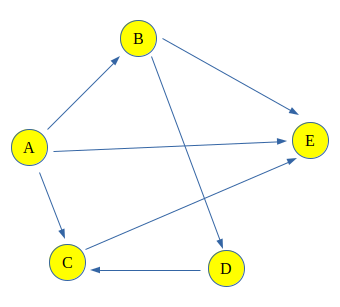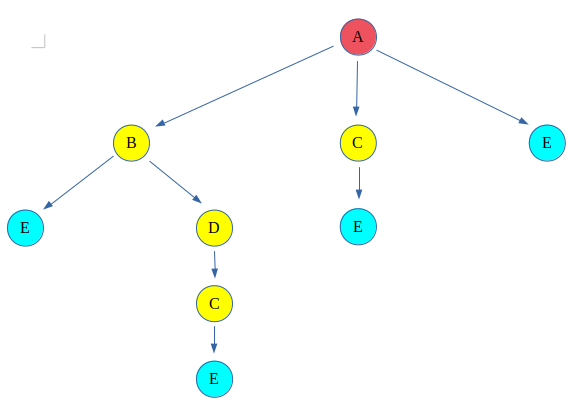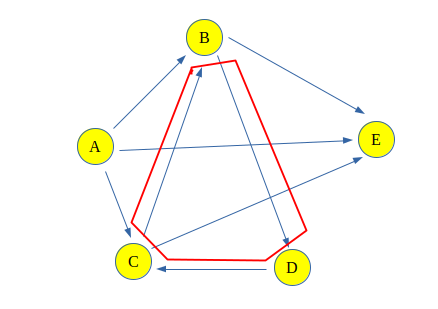# Count all possible paths between two vertices

Count the total number of ways or paths that exist between two vertices in a directed graph. These paths don’t contain a cycle, the simple enough reason is that a cycle contains an infinite number of paths and hence they create a problem.

Examples:

```For the following Graph:Input: Count paths between A and E
Output : Total paths between A and E are 4
Explanation: The 4 paths between A and E are:
A -> E
A -> B -> E
A -> C -> E
A -> B -> D -> C -> E

Input : Count paths between A and C
Output : Total paths between A and C are 2
Explanation: The 2 paths between A and C are:
A -> C
A -> B -> D -> C
```

## Recommended: Please try your approach on {IDE} first, before moving on to the solution.

Approach:
The problem can be solved using backtracking, that says take a path and start walking on it and check if it leads us to the destination vertex then count the path and backtrack to take another path. If the path doesn’t lead to the destination vertex, discard the path.
This type of graph traversal is called Backtracking.

Backtracking for above graph can be shown like this:
The red color vertex is the source vertex and the light-blue color vertex is destination, rest are either intermediate or discarded paths.This give four paths between source(A) and destination(E) vertex.

Why this solution will not work for a graph which contains cycles?
The Problem Associated with this is that now if one more edge is added between C and B, it would make a cycle (B -> D -> C -> B). And hence after every cycle through the loop, the length path will increase and that will be considered as a different path, and there would be infinitely many paths because of the cycle.Algorithm:

1. Create a recursive function that takes index of node of a graph and the destination index. Keep a global or a static variable count to store the count.
2. If the current nodes is the destination increase the count.
3. Else for all the adjacent nodes, i.e. nodes that are accessible from the current node, call the recursive function with the index of adjacent node and the destination.
4. Print the Count.

Implementation:

## C++

 `// C++ program to count all paths from a ` `// source to a destination. ` `#include ` ` `  `using` `namespace` `std; ` ` `  `// A directed graph using adjacency list ` `// representation ` `class` `Graph { ` ` `  `    ``// No. of vertices in graph ` `    ``int` `V; ` `    ``list<``int``>* adj; ` ` `  `    ``// A recursive function ` `    ``// used by countPaths() ` `    ``void` `countPathsUtil(``int``, ``int``, ``int``&); ` ` `  `public``: ` `    ``// Constructor ` `    ``Graph(``int` `V); ` `    ``void` `addEdge(``int` `u, ``int` `v); ` `    ``int` `countPaths(``int` `s, ``int` `d); ` `}; ` ` `  `Graph::Graph(``int` `V) ` `{ ` `    ``this``->V = V; ` `    ``adj = ``new` `list<``int``>[V]; ` `} ` ` `  `void` `Graph::addEdge(``int` `u, ``int` `v) ` `{ ` ` `  `    ``// Add v to u’s list. ` `    ``adj[u].push_back(v); ` `} ` ` `  `// Returns count of paths from 's' to 'd' ` `int` `Graph::countPaths(``int` `s, ``int` `d) ` `{ ` ` `  `    ``// Call the recursive helper ` `    ``// function to print all paths ` `    ``int` `pathCount = 0; ` `    ``countPathsUtil(s, d, pathCount); ` `    ``return` `pathCount; ` `} ` ` `  `// A recursive function to print all paths ` `// from 'u' to 'd'. visited[] keeps track of ` `// vertices in current path. path[] stores ` `// actual vertices and path_index is ` `// current index in path[] ` `void` `Graph::countPathsUtil(``int` `u, ``int` `d, ` `                           ``int``& pathCount) ` `{ ` `    ``// If current vertex is same as destination, ` `    ``// then increment count ` `    ``if` `(u == d) ` `        ``pathCount++; ` ` `  `    ``// If current vertex is not destination ` `    ``else` `{ ` `        ``// Recur for all the vertices adjacent to ` `        ``// current vertex ` `        ``list<``int``>::iterator i; ` `        ``for` `(i = adj[u].begin(); i != adj[u].end(); ++i) ` `            ``countPathsUtil(*i, d, pathCount); ` `    ``} ` `} ` ` `  `// Driver Code ` `int` `main() ` `{ ` ` `  `    ``// Create a graph given in the above diagram ` `    ``Graph g(5); ` `    ``g.addEdge(0, 1); ` `    ``g.addEdge(0, 2); ` `    ``g.addEdge(0, 3); ` `    ``g.addEdge(1, 3); ` `    ``g.addEdge(2, 3); ` `    ``g.addEdge(1, 4); ` `    ``g.addEdge(2, 4); ` ` `  `    ``int` `s = 0, d = 3; ` `    ``cout << g.countPaths(s, d); ` ` `  `    ``return` `0; ` `} `

## Java

 `// Java program to count all paths from a source ` `// to a destination. ` `import` `java.util.Arrays; ` `import` `java.util.Iterator; ` `import` `java.util.LinkedList; ` ` `  `// This class represents a directed graph using ` `// adjacency list representation ` ` `  `class` `Graph { ` ` `  `    ``// No. of vertices ` `    ``private` `int` `V; ` ` `  `    ``// Array of lists for ` `    ``// Adjacency List ` `    ``// Representation ` `    ``private` `LinkedList adj[]; ` ` `  `    ``@SuppressWarnings``(``"unchecked"``) ` `    ``Graph(``int` `v) ` `    ``{ ` `        ``V = v; ` `        ``adj = ``new` `LinkedList[v]; ` `        ``for` `(``int` `i = ``0``; i < v; ++i) ` `            ``adj[i] = ``new` `LinkedList<>(); ` `    ``} ` ` `  `    ``// Method to add an edge into the graph ` `    ``void` `addEdge(``int` `v, ``int` `w) ` `    ``{ ` ` `  `        ``// Add w to v's list. ` `        ``adj[v].add(w); ` `    ``} ` ` `  `    ``// A recursive method to count ` `    ``// all paths from 'u' to 'd'. ` `    ``int` `countPathsUtil(``int` `u, ``int` `d, ` `                       ``int` `pathCount) ` `    ``{ ` ` `  `        ``// If current vertex is same as ` `        ``// destination, then increment count ` `        ``if` `(u == d) { ` `            ``pathCount++; ` `        ``} ` ` `  `        ``// Recur for all the vertices ` `        ``// adjacent to this vertex ` `        ``else` `{ ` `            ``Iterator i = adj[u].listIterator(); ` `            ``while` `(i.hasNext()) { ` `                ``int` `n = i.next(); ` `                ``pathCount = countPathsUtil(n, d, pathCount); ` `            ``} ` `        ``} ` `        ``return` `pathCount; ` `    ``} ` ` `  `    ``// Returns count of ` `    ``// paths from 's' to 'd' ` `    ``int` `countPaths(``int` `s, ``int` `d) ` `    ``{ ` ` `  `        ``// Call the recursive method ` `        ``// to count all paths ` `        ``int` `pathCount = ``0``; ` `        ``pathCount = countPathsUtil(s, d, ` `                                   ``pathCount); ` `        ``return` `pathCount; ` `    ``} ` ` `  `    ``// Driver Code ` `    ``public` `static` `void` `main(String args[]) ` `    ``{ ` `        ``Graph g = ``new` `Graph(``5``); ` `        ``g.addEdge(``0``, ``1``); ` `        ``g.addEdge(``0``, ``2``); ` `        ``g.addEdge(``0``, ``3``); ` `        ``g.addEdge(``1``, ``3``); ` `        ``g.addEdge(``2``, ``3``); ` `        ``g.addEdge(``1``, ``4``); ` `        ``g.addEdge(``2``, ``4``); ` ` `  `        ``int` `s = ``0``, d = ``3``; ` `        ``System.out.println(g.countPaths(s, d)); ` `    ``} ` `} ` ` `  `// This code is contributed by shubhamjd. `

## Python3

 `# Python 3 program to count all paths  ` `# from a source to a destination.  ` ` `  `# A directed graph using adjacency  ` `# list representation  ` `class` `Graph: ` ` `  `    ``def` `__init__(``self``, V): ` `        ``self``.V ``=` `V  ` `        ``self``.adj ``=` `[[] ``for` `i ``in` `range``(V)] ` `     `  `    ``def` `addEdge(``self``, u, v): ` `         `  `        ``# Add v to u’s list.  ` `        ``self``.adj[u].append(v) ` `     `  `    ``# Returns count of paths from 's' to 'd'  ` `    ``def` `countPaths(``self``, s, d): ` `         `  `        ``# Mark all the vertices  ` `        ``# as not visited  ` `        ``visited ``=` `[``False``] ``*` `self``.V ` `     `  `        ``# Call the recursive helper  ` `        ``# function to print all paths  ` `        ``pathCount ``=` `[``0``]  ` `        ``self``.countPathsUtil(s, d, visited, pathCount)  ` `        ``return` `pathCount[``0``] ` `     `  `    ``# A recursive function to print all paths  ` `    ``# from 'u' to 'd'. visited[] keeps track   ` `    ``# of vertices in current path. path[]  ` `    ``# stores actual vertices and path_index  ` `    ``# is current index in path[]  ` `    ``def` `countPathsUtil(``self``, u, d,  ` `                       ``visited, pathCount): ` `        ``visited[u] ``=` `True` `     `  `        ``# If current vertex is same as  ` `        ``# destination, then increment count  ` `        ``if` `(u ``=``=` `d): ` `            ``pathCount[``0``] ``+``=` `1` `     `  `        ``# If current vertex is not destination  ` `        ``else``: ` `             `  `            ``# Recur for all the vertices  ` `            ``# adjacent to current vertex ` `            ``i ``=` `0` `            ``while` `i < ``len``(``self``.adj[u]): ` `                ``if` `(``not` `visited[``self``.adj[u][i]]):  ` `                    ``self``.countPathsUtil(``self``.adj[u][i], d,  ` `                                        ``visited, pathCount) ` `                ``i ``+``=` `1` `     `  `        ``visited[u] ``=` `False` ` `  `# Driver Code  ` `if` `__name__ ``=``=` `'__main__'``: ` ` `  `    ``# Create a graph given in the  ` `    ``# above diagram  ` `    ``g ``=` `Graph(``4``)  ` `    ``g.addEdge(``0``, ``1``)  ` `    ``g.addEdge(``0``, ``2``)  ` `    ``g.addEdge(``0``, ``3``)  ` `    ``g.addEdge(``2``, ``0``)  ` `    ``g.addEdge(``2``, ``1``)  ` `    ``g.addEdge(``1``, ``3``)  ` ` `  `    ``s ``=` `2` `    ``d ``=` `3` `    ``print``(g.countPaths(s, d)) ` ` `  `# This code is contributed by PranchalK `

## C#

 `// C# program to count all paths from a source ` `// to a destination. ` `using` `System; ` `using` `System.Collections.Generic; ` ` `  `// This class represents a directed graph using ` `// adjacency list representation ` `public` `class` `Graph { ` ` `  `    ``// No. of vertices ` `    ``int` `V; ` ` `  `    ``// Array of lists for ` `    ``// Adjacency List ` `    ``// Representation ` `    ``private` `List<``int``>[] adj; ` ` `  `    ``Graph(``int` `v) ` `    ``{ ` `        ``V = v; ` `        ``adj = ``new` `List<``int``>[v]; ` `        ``for` `(``int` `i = 0; i < v; ++i) ` `            ``adj[i] = ``new` `List<``int``>(); ` `    ``} ` ` `  `    ``// Method to add an edge into the graph ` `    ``void` `addEdge(``int` `v, ``int` `w) ` `    ``{ ` ` `  `        ``// Add w to v's list. ` `        ``adj[v].Add(w); ` `    ``} ` ` `  `    ``// A recursive method to count ` `    ``// all paths from 'u' to 'd'. ` `    ``int` `countPathsUtil(``int` `u, ``int` `d, ` `                       ``int` `pathCount) ` `    ``{ ` ` `  `        ``// If current vertex is same as ` `        ``// destination, then increment count ` `        ``if` `(u == d) { ` `            ``pathCount++; ` `        ``} ` ` `  `        ``// Recur for all the vertices ` `        ``// adjacent to this vertex ` `        ``else` `{ ` `            ``foreach``(``int` `i ``in` `adj[u]) ` `            ``{ ` `                ``int` `n = i; ` `                ``pathCount = countPathsUtil(n, d, ` `                                           ``pathCount); ` `            ``} ` `        ``} ` `        ``return` `pathCount; ` `    ``} ` ` `  `    ``// Returns count of ` `    ``// paths from 's' to 'd' ` `    ``int` `countPaths(``int` `s, ``int` `d) ` `    ``{ ` ` `  `        ``// Call the recursive method ` `        ``// to count all paths ` `        ``int` `pathCount = 0; ` `        ``pathCount = countPathsUtil(s, d, ` `                                   ``pathCount); ` `        ``return` `pathCount; ` `    ``} ` ` `  `    ``// Driver Code ` `    ``public` `static` `void` `Main(String[] args) ` `    ``{ ` `        ``Graph g = ``new` `Graph(5); ` `        ``g.addEdge(0, 1); ` `        ``g.addEdge(0, 2); ` `        ``g.addEdge(0, 3); ` `        ``g.addEdge(1, 3); ` `        ``g.addEdge(2, 3); ` `        ``g.addEdge(1, 4); ` `        ``g.addEdge(2, 4); ` ` `  `        ``int` `s = 0, d = 3; ` `        ``Console.WriteLine(g.countPaths(s, d)); ` `    ``} ` `} ` ` `  `// This code is contributed by Rajput-Ji `

Output:

`3`

Complexity Analysis:

• Time Complexity: O(N!).
If the graph is complete then there can be around N! recursive calls, so the time Complexity is O(N!)
• Space Complexity: O(1).
Since no extra space is required.

Attention reader! Don’t stop learning now. Get hold of all the important DSA concepts with the DSA Self Paced Course at a student-friendly price and become industry ready.

My Personal Notes arrow_drop_upCheck out this Author's contributed articles.

If you like GeeksforGeeks and would like to contribute, you can also write an article using contribute.geeksforgeeks.org or mail your article to contribute@geeksforgeeks.org. See your article appearing on the GeeksforGeeks main page and help other Geeks.

Please Improve this article if you find anything incorrect by clicking on the "Improve Article" button below.

Article Tags :
Practice Tags :

33

Please write to us at contribute@geeksforgeeks.org to report any issue with the above content.